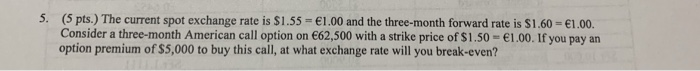# 5. (5 pts.) The current spot exchange rate is \$1.55 1.00 and the three-month forward rate is \$1.6...5. (5 pts.) The current spot exchange rate is \$1.55 1.00 and the three-month forward rate is \$1.60 1.00. Consider a three-month American call option on €62.500 with a strike price of S 1.50·ei .00, if you pay an option premium of \$5,000 to buy this call, at what exchange rate will you break-even?

Breakeven exchange rate is given as equal to

=5000/62500+1.50=1.58\$/€

##### Add Answer of: 5. (5 pts.) The current spot exchange rate is \$1.55 1.00 and the three-month forward rate is \$1.6...
More Homework Help Questions Additional questions in this topic.

• #### A catering company has 15 customer orders during its first month, for each month afterward, the comopany has double the number of orders than the previous month

Need Online Homework Help?